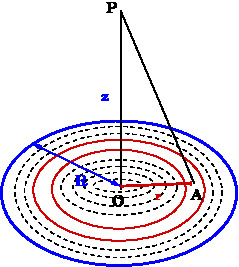# [YMP/EM-02006] Electric Field due to a Uniformly Charged Disk

For page specific messages
For page author info

We compute the electric field  due to a uniformly charged disk at a point on the axis of the disk.

The surface charge density of the disc  $\sigma$ is given to be uniform. First divide the disc  into several concentric rings and consider one such ring of radius $$r$$ and thickness $$dr$$. Since the surface charge density is given to be uniform, the only the $$z-$$ component of  electric field at point $$P$$ will be non zero.  The   area of the ring is $$2\pi r dr$$ and hence the charge of this ring is $$dq = 2\pi r\, dr \sigma$$. The $$z-$$ component of the electric field at point $$P$$ can now be written.  Thus we have
\begin{eqnarray}
\vec{E}_z=\frac{dq z}{4\pi\epsilon_0(z^2+r^2)^{3/2}} \label{eq10}
\end{eqnarray}
If the radius of the disc is $$a$$ and the total charge is $$Q$$, we get
$$Q = \sigma (\pi a^2) \Rightarrow \sigma = \frac{Q}{\pi a^2}.$$
Therefore $dq=\sigma (2\pi r dr)=\frac{Q}{\pi a^2}(2\pi r dr) = (2Qr dr)/a^2$.  Using the result for the field due to ring we get\begin{eqnarray}\nonumber\vec{E}_z&=&\frac{2Qz}{a^2 4\pi\epsilon_0}\int_0^a\frac{rdr}{(z^2+r^2)^{3/2}}\\\nonumber&=&\frac{2Qz}{a^2 4\pi\epsilon_0}\Big[-(z^2+r^2)^{-1/2}\Big]_{r=0}^{r=a}\\\nonumber&=&\frac{2Qz}{a^2 4\pi\epsilon_0}\Big[-(z^2+a^2)^{-1/2}+\frac{1}{z}\Big]\\&=&\frac{2Qz}{a^2 4\pi\epsilon_0}\Big[1-\frac{z}{(z^2+a^2)^{1/2}}\Big]\\ \label{eq11}\end{eqnarray}

For $z>>a$, we use
\begin{equation}
\frac{z}{(z^2+a^2)^{-1/2}} = \big(1+(z/a)^2)^{-1/2}\big) = 1 - \frac{1}{2}\frac{a^2}{z^2} + \ldots,
\end{equation}
in \eqref{eq11} and we get the expected result
\begin{eqnarray}
{E}&\approx&\Big(\frac{2Q}{4\pi\epsilon_0a^2}\Big)\times\frac{a^2}{2z^2}=\frac{Q}{4\pi\epsilon_0z^2} \label{eq12}
\end{eqnarray}

### Exclude node summary :

n

4727:Diamond Point

0

X